# CDS 2011 Maths Question Paper -2

1. The least number which when divided by 5, 6, 7 and 8 leaves a remainder 3 is
• 423
• 843
• 1683
• 2523
2. Two numbers are in the ratio 3 : 5. If 9 is subtracted from each number, then they are in the ratio of 12 : 23. What is the second number ?
• 44
• 55
• 66
• 77
1. What is the HCF of the polynomials x4 – 3x + 2, x3 – 3x2 + 3x – I and x4 – 1 ?
• x – 1
• x + 1
• x2 – 1
• None of the above
2. If 5x-7y + 10/1= 3x + 2y+1/8= 11x + 4y-10/9 then what is x + y equal to ?
• 1
• 2
• 3
• -3
3. A certain sum at simple interest amounts to Rs. 1350 in 5 years and to Rs. 1620 in 8 years. What is the sum ?
• 700
• 800
• 900
• 1000
4. What is the number of circles which pass through three given points not in a straight line ?
• 0
• 1
• 2
• 3
1. A cylindrical tank 7 m in diameter, contains water to a depth of 4 m. What is the total area of wetted surface ?
• 110 m2
• 5 m2
• 5 m2
• 5 m2

2. A wall is of the form of a trapezium with height 4 m and parallel sides being 3 m and 5 m. What is the cost of painting the wall, if the rate of painting is Rs. 25/- per square metre ?.
• 240
• 400
• 480
• 800
3. A lawn 30 m long and 16 m wide is surrounded by a path 2 m wide. What is the area of the path ?
• 200 m2
• 280 m2
• 300 m2
• 320 m2
4. The angle of elevation of the top of an incomplete vertical pillar at a horizontal distance of 100 m from its base is 45°. If the angle of elevation of the top of complete pillar at the same point is to be 60°, then the height of the incomplete pillar is to be increased by
• 50 √2m
• 100 m
• 100 ( √3- 1) m
• 100 ( √3+ 1) m
1. The system of equations x + 2y = 3 and 3x + 6y = 9 has
• unique solution
• no solution
• infinitely many solutions
• finite number of solutions
2. A hollow sphere of internal and external diameters 4 cm and 8 cm respectively is melted into a cone of base diameter 8 cm. What is the height of the cone ?
• 12 cm
• 14 cm
• 17 cm
• 18 cm
1. The sum of digits of a two digit number is 8 and the difference between the number and that formed by reversing the digits is 18. What is the difference between the digits of the number ?
• 1
• 2
• 3
• 4
2. A fruit-seller buys lemons at 2 for a rupee and sells them at 5 for three rupees. What is his gain percent ?
• 10%
• 15%
• 20%
• 25%
3. A man sold two watches, each for Rs. 495. If he gained 10% on one watch and suffered a loss of 10% on the other, then what is the loss or gain percentage in the transaction ?
• 1% gain
• 1% loss
• 100/99% loss
• No gain no loss
4. If 6≤ x ≤ 8,then which following is correct?
• (x – 6) (x -8) ≥0
• (x – 6) (x -8) >0
• (x – 6) (x – 8) ≤0
• (x – 6) (x ‘ 8) <0
1. What is the smallest number that must be added to 1780 to make it a perfect square ?
• 39
• 49
• 59
• 69
2. What are the roots of the quadratic equation a2b2x2 – (a2 + b2) x + 1 = 0 ?
• 1/a2,1/b2
• -1/a2,-1/b2
• 1/a2,-1/b2
• -1/a2,1/b2
1. A cylindrical vessel of height 10 cm has base radius 60 cm. If d is the diameter of a spherical vessel of equal volume, then what is d equal to ?
• 30 cm
• 60 cm
• 90 cm
• 120 cm
2. The surface area of a sphere is 616 square cm. If its radius is changed so that the area gets reduced by 75%, then the radius becomes
• 6 cm
• 3 cm
• 5 cm
• 5 cm
3. From a lighthouse the angles of depression of two ships on opposite sides of the light- house are observed to be 30° and 45°. If the height of lighthouse is h, what is the distance between the ships ?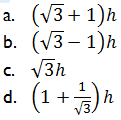1. If (x, y) = (4, 1) is the solution of the pair of linear equations mx + y = 2x + ny = 5, then what is m + n equal to ?
• -2
• -I
• 2
• 1
1. What is the value of (2.3)3-0.027/ (2.3)2 +0.69 + 0.09?
• 3
• 3
• 1
• 2
1. The earth takes 24 hours to rotate about its own axis. Through what angle will it turn in 4 hours and 12 minutes ?
• 63°
• 64°
• 65°
• 70°
2. An employee is required to contribute 10% of his pay to General Provident Fund. If he gets Rs. 13,500 as net pay in a month, then what is the monthly General Provident Fund contribution (assuming no other deductions) ?
• 1215
• 1350
• 1500
• 1650
3. If Rs. 8400 is divided among A, B and C in the ratio 1/5:1/6:1/10 , what is the share of A ?
• 1800
• 3000
• 3600
• 4000
4. If P : Q = 3/5:5/7, Q : R =3/4:2/5, then what is P : Q : R equal to ?
• 3/5:5/7:2/5
• 9/20:15:28:2/7
• 3/5:3/4:2/5
• 3/5:5/7:3/4
1. How many men will be required to plough 100 acres of land in 10 days if 10 men require 8 days to plough 20 acres of land ?
• 30
• 40
• 50
• 60
1. A train 280 m long is moving at a speed of 60 kmph. What is the time taken by the train to cross a platform 220 m long ?
• 45 s
• 40 s
• 35 s
• 30 s
1. If x + y + z = 0, then what is xyz /(x + y)(y + z)(z+ x) equal to ?
[x ≠ – y, y ≠ – z, z ≠ – x]
• -1
• 1
• xy + yz + zx
• None of the above
1. What is the remainder when (x11 + 1) is divided by (x + 1) ?
• 0
• 2
• 11
• 12
2. If (x – 3) is a factor of (x2 + 4px – lip), then what is the value of p ?
• -9
• -3
• – 1
• 1
3. What is the LCM of a3b – ab3, a3b2 + a2b3 and ab(a + b) ?
• a2b2(a2 – b2)
• ab(a2 – b2)
• a2b2 + ab3
• a3b3(a2 – b2)
4. A circle circumscribes a rectangle with sides 16 cm and 12 cm. What is the area of the circle ?
• 48π square cm
• 50π square cm
• 100π square cm
• 200π square cm
1. What is the area of an equilateral triangle having altitude equal to 2√3 cm ?
• √3 square cm
• 2√3 square cm
• 3√3 square cm
• 4√3 square cm
2. The point of concurrence of the altitudes of a triangle is called
• Circum-centre
• Ortho-centre
• In-centre
• Centroid
3. If sin θ/ cos θ + cos θ /sinθ  = 2 with 0 < θ < 90°, then what is θ equal to ?
• 30°
• 45°
• 60°
• 75°
1. If the population figures are given for each State of India, then the data can be classified as
• Qualitative
• Quantitative
• Chronological
• Geographical
2. A student moves √2x km east from his residence and then moves x km north. He then goes x km north-east and finally he takes a turn of 90° towards right and moves a distance x km and reaches his school. What is the shortest distance of the school from his residence ?
• (2 √2+ l)x km
• 3x km
• 2√2 x km
• 3√2 x km
3. The lengths of two sides of a right angled triangle which contain the right angle are ‘a’ and ‘b’ respectively. Three squares are drawn on the three sides of the triangle on the outer side. What is the total area of the triangle and the three squares ?
• 2(a2 + b2) + ab
• 2(a2 + b2) + 2.5ab
• 2(a2 + b2) + 0.5ab
• 5(a2 + b2)
4. What is 3.76 – 1.4576  equal to ?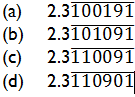5. The sum of two numbers is 10 and their product is 20. What is the sum of their reciprocals ?
• 1/10
• 1/2
• 1
• 2
6. What is x2-3x+2/x2 -5x + 6 x2 -5x + 4/ x2 -7x + 12 equal to?
• x+3/x-3
• 1
• x+1/x-1
• 2
1. From a solid cylinder of height 4 cm and radius 3 cm, a conical cavity of height 4 cm and of base radius 3 cm is hollowed out. What is the total surface area of the remaining solid ?
• 15π square cm
• 22π square cm
• 33π square cm
• 48π square cm
1. If A = π/6 and B = π/3, then which of the following is/are correct ?
2. sin A + sin B = cos A + cos B
3. tan A + tan B = cot A + cot B

Select the correct answer using the code given below :

• 1 only
• 2 only
• Both 1 and 2
• Neither 1 nor 2
1. If a + b + c = 6, a2 + b2 + c2 = 26, then what is ab + be + ca equal to ?
• 0
• 2
• 4
• 5
2. Which among the following is the largest four digit number that is divisible by 88 ?
• 9988
• 9966
• 9944
• 8888
3. A, B, C can do a piece of work individually in 8, 10 and 15 days respectively. A and B start working but A quits after working for 2 days. After this, C joins B till the completion of work. In how many days will the work be completed ?
• 53/9 days
• 34/7 days
• 85/13 days
• 53/10 days
4. The radii of the circular ends of a bucket of height 40 cm are of lengths 35 cm and 14 cm. What is the volume of the bucket ?
• 60060 cubic cm
• 70040 cubic cm
• 80080 cubic cm
• 80160 cubic cm
1. If S is the total surface area of a cube and V is its volume, then which one of the following is correct ?
• V3 = 216 S2
• S3 = 216 V2
• S3 = 6V2
• S2 = 36V3

1. If A, B, C, D are the successive angles of a cyclic quadrilateral, then what is cos A + cos B + cos C + cos D equal to ?
• 4
• 2
• 1
• 0
2. A number when divided by 2, 3 or 5 gives remainder 1. The number is
• 31
• 47
• 49
• 53
3. Let the observations at hand be arranged in increasing order. Which one of the following measures will not be affected when the smallest and the largest observations are removed ?
• Mean
• Median
• Mode
• Standard deviation
4. If sinθ and cosθ  are the roots of the equation ax2 – bx + c = 0, then which one of the following is correct ?
• a2 + b2 + 2ac = 0
• a2 – b2 + 2ac = 0
• a2 + c2 + 2ab = 0
• a2 – b2 – 2ac = 0
5. A unit radian is approximately equal to
• 57° 17’43”
• 57° 17′ 45″
• 57° 17′ 47″
• 57° 17’49”
6. The arithmetic mean and geometric mean of two numbers are 14 and 12 respectively. What is the harmonic mean of the numbers ?
• 10
• 13
• 32/3
• 72/7
7. Let A and B be two points. What is the locus of the point P such that angle APB = 90° ?
• the line AB itself
• the point P itself
• the circumference of the circle with AB as diameter
• the line perpendicular to AB and bisecting AB
8. 76 ladies complete a job in 33 days. Due to some reason some ladies did not join the work and therefore it was completed in 44 days. The number of ladies who did not report for the work is
• 17
• 18
• 19
• 20
1. If 3x3 – 2x2y -13xy2 + 10y3 is divided by x – 2y, then what is the remainder ?
• 0
• x
• y + 5
• x – 3
1. The angles of elevation of the top of a tower from two points situated at distances 36 m and 64 m from its base and in the same straight line with it are complementary. What is the height of the tower ?
• 50 m
• 48 m
• 25 m
• 24 m
2. A grassy field has the shape of an equilateral triangle of side 6 m. A horse is tied to one of its vertices with a rope of length 4-2 m. The percentage of the total area of the field which is available for grazing is best approximated by
• 50%
• 55%
• 59%
• 62%
3. What is the value of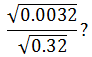• 0001
• 001
• 01
• 1
4. Consider the following statements :
5. Set of points of a given line is a finite set.
6. Intelligent students in a class are a set.
7. Good books in a school library are a set.

Which of the above statements is/are not correct ?

• 1 only
• 2 and 3 only
• 1 and 2 only
• 1, 2 and 3
1. If α and β are the roots of the equation x2 – 6x + 6 = 0, what is α3 + β3 + α2 + β2 + α+ β equal to ?
• 150
• 138
• 128
• 124
2. If 2m + 21+ m = 24, then what is value of m ?
• 0
• 1/3
• 3
• 6
3. If n is a natural number, then √n is
• always a natural number
• always a rational number
• always an irrational number
• either a natural number or an irrational Number
4. The frequency distribution of a variate X is as given below :
 X 2 3 4 5 6 frequency 2 7 14 22 30

What is the cumulative frequency of variate X = 5 ?

• 36
• 44
• 45
• 52
1. Consider the following statements in respect of the set S = {1, 2, 3, ……,n} :
2. (n + 1 )/2 is the median of the numbers in S.
3. n is the mode of the numbers in S.

Which of the above statements is/are correct ?

• 1 only
• 2 only
• Both 1 and 2
• Neither 1 nor 2
1. The radii of two cylinders are in the ratio 2 : 3 and their curved surface areas are in the ratio 5 : 3. What is the ratio of their volumes ?
• 20 : 27
• 10 : 9
• 9 : 10
• 27 : 20
2. The areas of two circles are in the ratio 1 : 2. If the two circles are bent in the form of squares, what is the ratio of their areas ?
• 1:2
• 1 : 3
• 1 :√2
• 1:4
3. The area of a rectangle whose length is 5 units more than twice its width is 75 square units. What is its width ?
• 3 units
• 5 units
• 7 units
• 10 units
4. If the diameter of a sphere is doubled, how does its surface area change ?
• It increases two times.
• It increases three times.
• It increases four times,
• It increases eight times.
5. The sum which amounts to Rs. 364.80 in 8 years at 3.5% simple interest per annum is :
• 285
• 280
• 275
• 270
1. The length of shadow of a tree is 16 m when the angle of elevation of the sun is 60°. What is the height of the tree ?
• 8 m
• 16 m
• 16 √3 m
• 16/ √3 m
2. A sphere is inscribed in a cubical box such that the sphere is tangent to all six faces of the box. What is the ratio of the volume of the cubical box to the volume of sphere?
• 36π
• 4π /3
• 6/π
1. The product of two numbers is 6912 and their GCD is 24. What is their LCM ?
• 280
• 286
• 288
• 296
2. The area of a triangle ABC is equal to area of square of side length 6 cm. What is the length of the altitude to AB where AB = 9 cm ?
• 18 cm
• 14 cm
• 12 cm
• 8 cm
3. What is the LCM of 2/3,7/9,14/15?
• 7/3
• 14/3
• 2/3
• 1/3
1. A car travels along the four sides of a square at speeds v, 2v, 3v, 4v respectively. If u is the average speed of the car in its travel around the square, then which one of the following is correct ?
• u = 2.25v
• u = 3v
• v < u < 2v
• 3v < u < 4v
2. What is the number of wax balls, each of radius 1 cm, that can be molded out of a sphere of radius 8 cm ?
• 256
• 512
• 768
• 1024
3. 10 cylindrical pillars of a building have to be painted. The diameter of each pillar is 70 cm and the height is 4 m. What is the cost of painting at the rate of Rs. 5 per square metre ?
• 400
• 440
• 480
• 500
1. Consider the following statements :
2. The product of any three consecutive integers is divisible by 6.
3. Any integer can be expressed in one of the three forms 3k, 3k + 1, 3k + 2 where k is an integer.

Which of the above statements is/are correct ?

• I only
• 2 only
• ‘both 1 and 2
• Neither 1 nor 2
1. The fourth proportional to 7, 11, 14 is
• 16
• 18
• 20
• 22
2. ABC is an isosceles triangle such that AB = BC = 8 cm and ∠ABC = 90°. What is the length of the perpendicular drawn from B on AC ?
• 4 cm
• 4√2 cm
• 2√2  cm
• 2 cm
1. What is the length of the perpendicular drawn from the centre of circle of radius r on the chord of length √3 r ?
• r
• √2 r
• r/2
• r/4
2. If α and β are complementary angles, then what is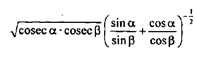equal to ?
• 0
• 1
• 2
• None of the above
3. Consider the following statements ;
4. The opposite angles of a cyclic quadrilateral are supplementary.
5. Angle subtended by an arc at the centre is double the angle subtended by it at any point on the remaining part of the circle.

Which one of the following is correct in respect of the above statements ?

• Statement-1=> statement-2
• Statement-2 => statement-1
• Statement-1 statement-2
• Neither statement-1 => statement-2 nor statement-2 => statement-1
1. Which one of the following is a prime number ?
• 161
• 171
• 173
• 221
2. What is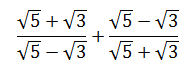equal to ?
• 16
• 8
• 4
• √15
3. What is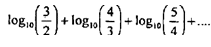up to 8 terms equal to ?
• 0
• 1
• log105
• None of the above
4. In the figure given above, ∠B = 38°, AC = BC and AD = CD. What is D equal to ?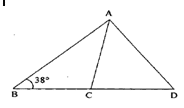• 26°
• 28°
• 38°
• 52°
5. In the figure given above, LM is parallel to QR. If LM divides the triangle PQR such that area of trapezium LMRQ is two times the area of triangle PLM, then what is PL/PQ equal to ?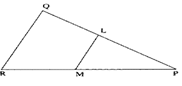• 1/√2
• 1/√3
• ½
• 1/3
1. The largest integer that divides product of any four consecutive integers is
• 4
• 6
• 12
• 24
1. In the figure given above, AB is parallel to CD. if ∠DCE =x and ∠ABE=y, than what is ∠CEB equal to?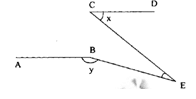• y-x
• (x+y)/2
• x+y-( /2)
• x+y –
1. In the figure given above, AB is parallel to CD. If ∠BAF = 98° and ∠AFC = 144°, then what is ∠ECD equal to ?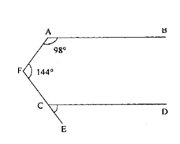• 62°
• 64°
• 82°
• 84°
2. How many degrees are there in an angle which equals two-third of its complement ?
• 36°
• 45°
• 48°
• 60°
3. In the figure given above, PQ is parallel to RS. What is ∠NMS equal to ?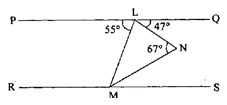• 20°
• 23°
• 27°
• 47°
4. In the figure given above, O is the centre of the circle, OA = 3 cm, AC = 3 cm and OM is perpendicular to AC. What is ∠ABC equal to ?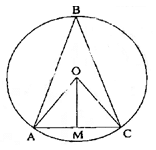• 60°
• 45°
• 30°
• None of the above
5. AC is the diameter of the circum-circle of the cyclic quadrilateral ABCD. If ∠BDC = 42°, then what is ∠ACB equal to ?
• 42°
• 45°
• 48° .
• 58°
1. The diameter of a circle circumscribing a square is 15√2 What is the length of the side of the square ?
• 15 cm
• 12 cm
• 10 cm
• 5 cm